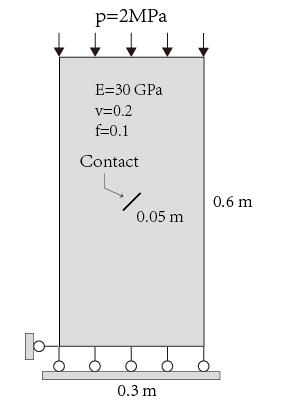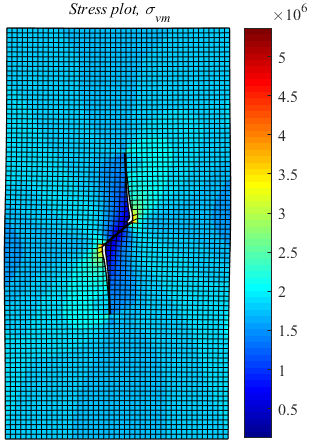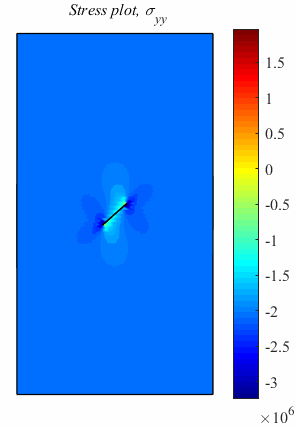PhiPsi

Example 1: Propagation and Intersection of two cracks under tensile load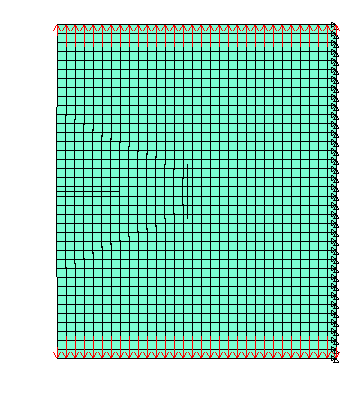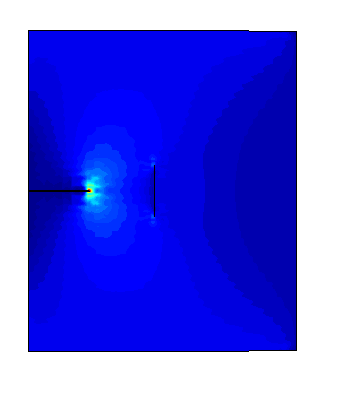Example 2: Multi-cracks propagation under tensile load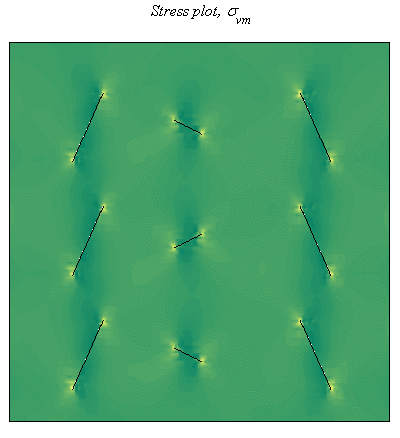Example 3: Crossing cracks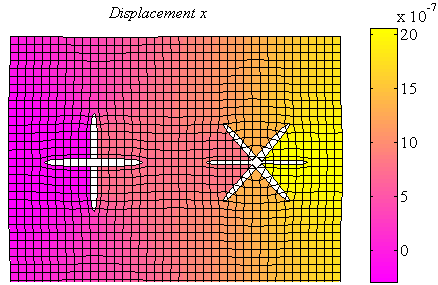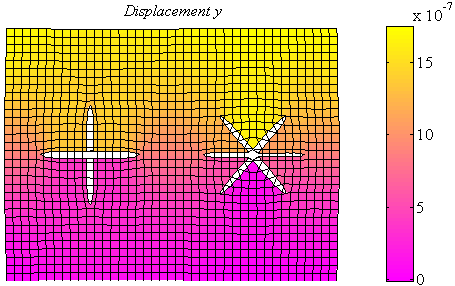Example 4: Cracks meet holes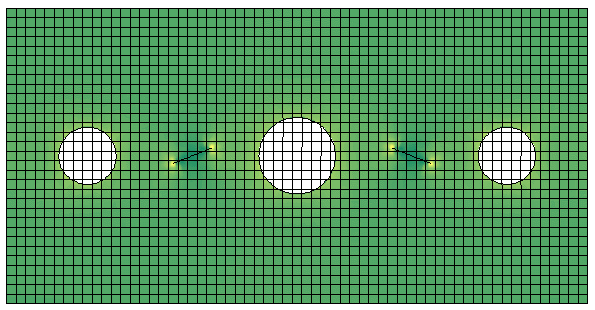Example 5: Propogation of cracks emerged from randomly generated holes under tensile load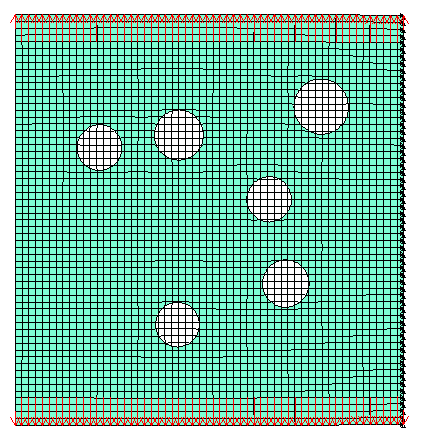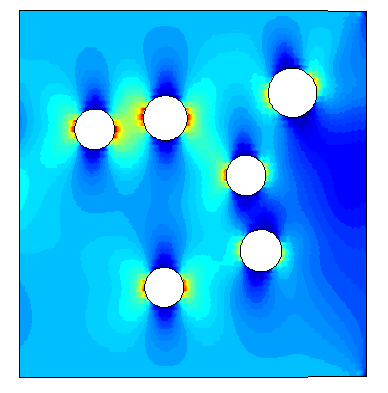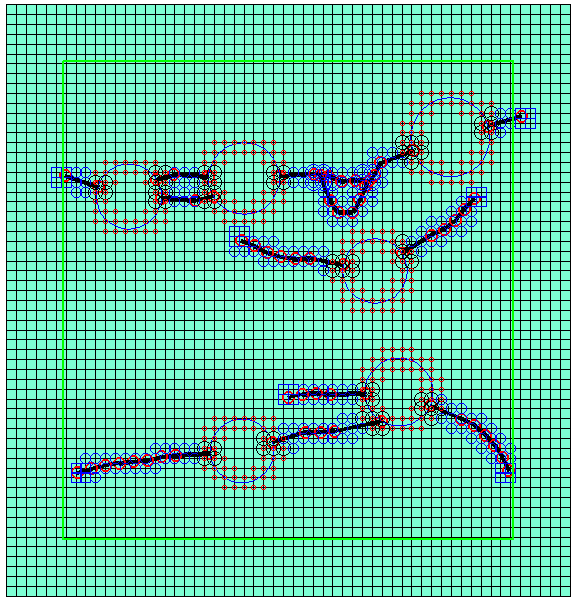Example 6: Deformation of composite beam containing randomly generated inclusions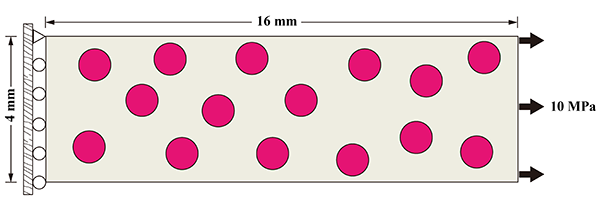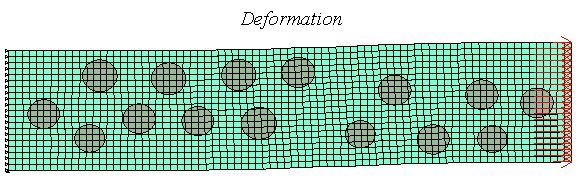Example 7: Hydraulic fracturing simulation： single hydraulic crack without in-situ stress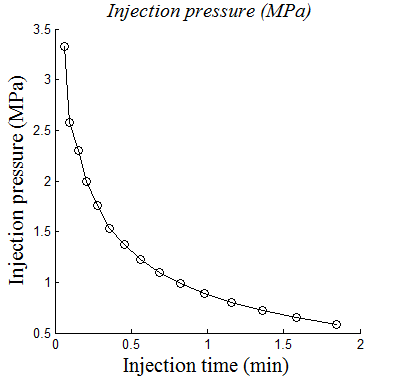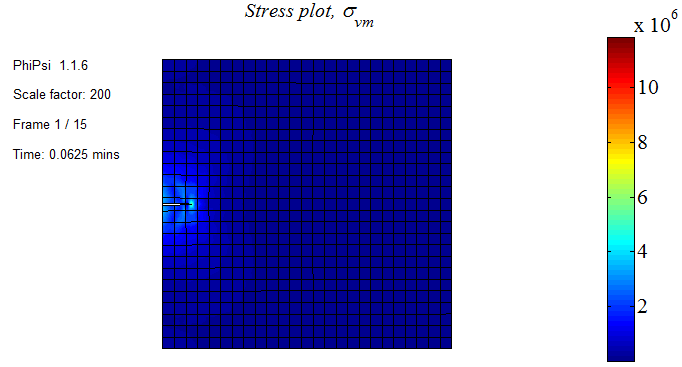Example 8: Hydraulic fracturing simulation: hydraulic crack meets frictional natural crack (in-situ stresses are 3MPa and 5MPa)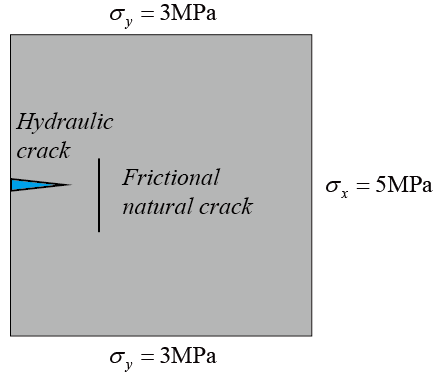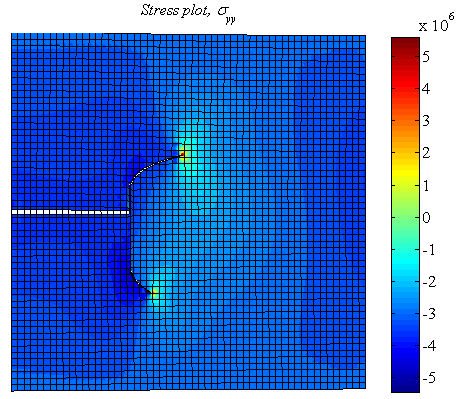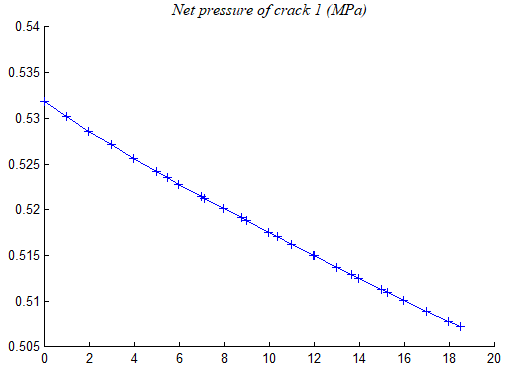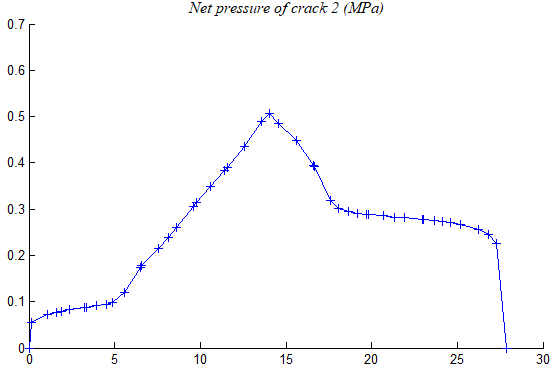Example 9: Destruction of a dam under the action of earthquake acceleration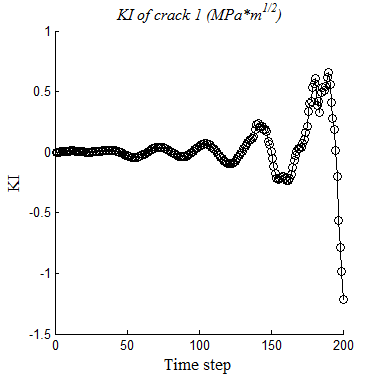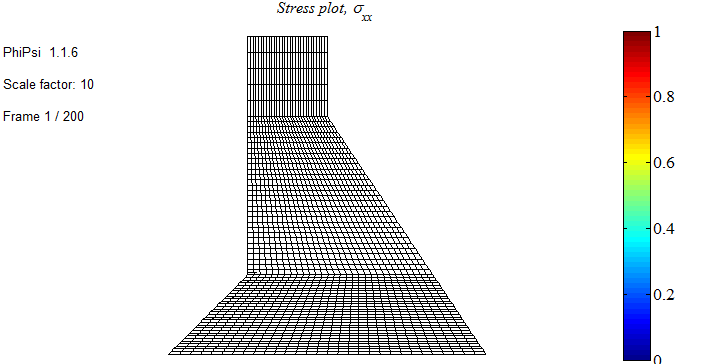Example 10: Nonlinear analysis - Plastic deformation (Von Mises)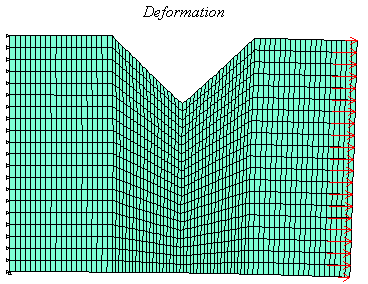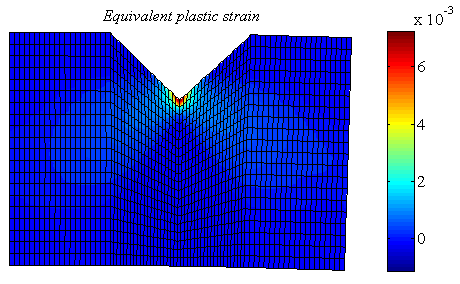Example 11: Three-dimensional crack of a brick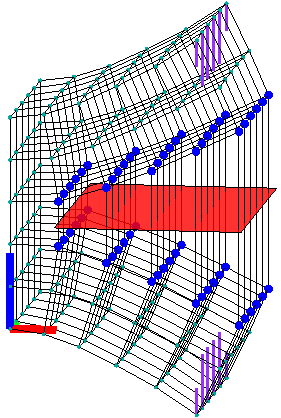Example 12: Three-dimensional crack of a hollow cylinder under high internal pressure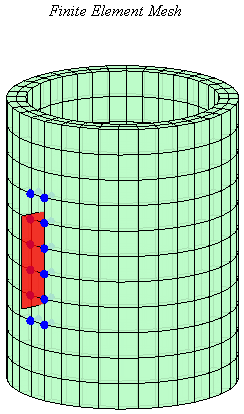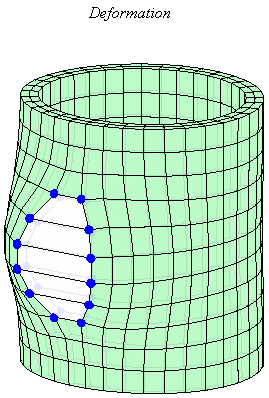Example 13: Propagation of a three-dimensional rectangle crack under tensile load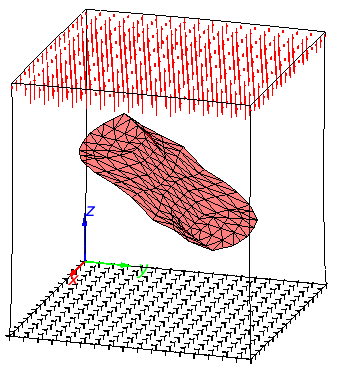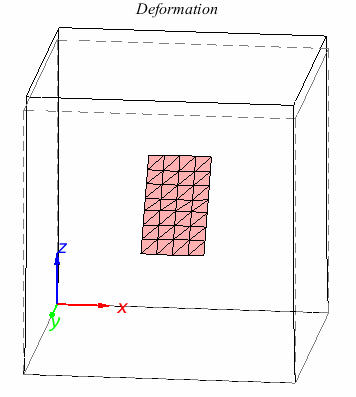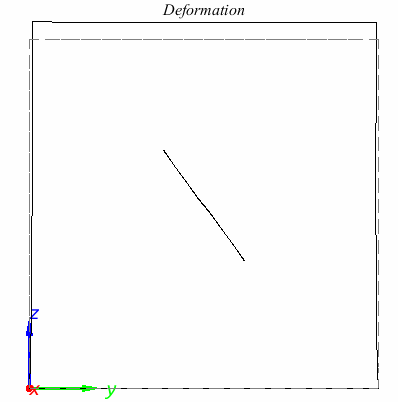Example 14: Propagation of two three-dimensional circular cracks under tensile load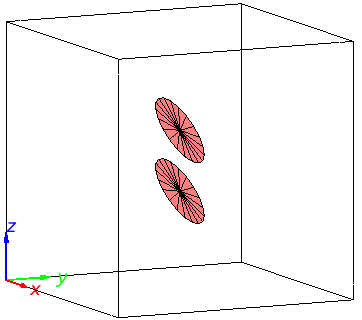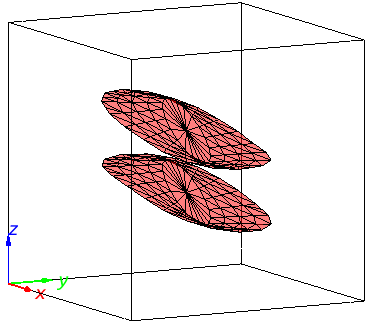Example 15: Temperature distribution of a square plate with adiabatic cracks and hole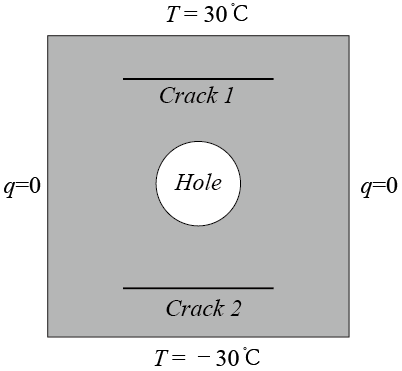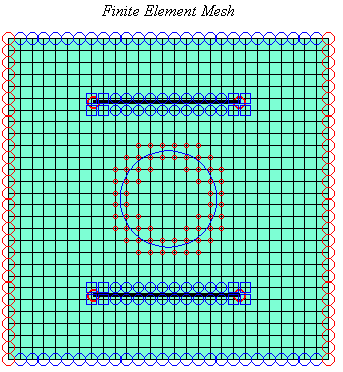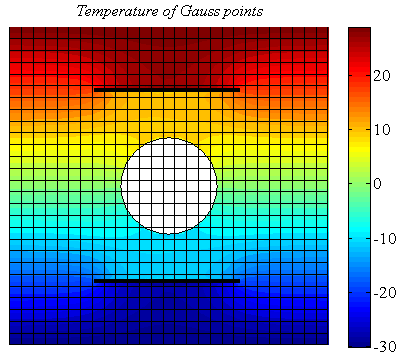Example 16: Propagation of a 2D crack caused by the excavation of the rock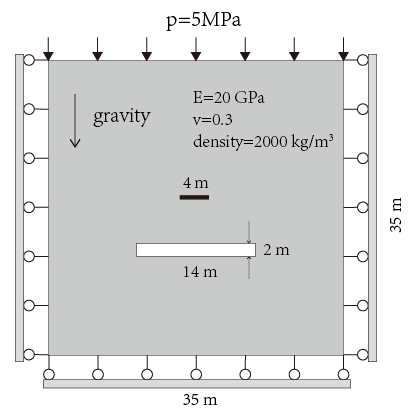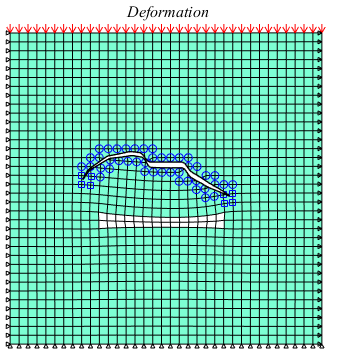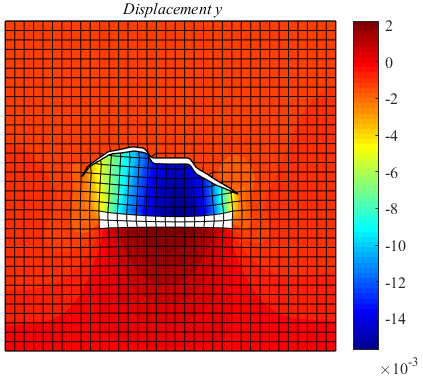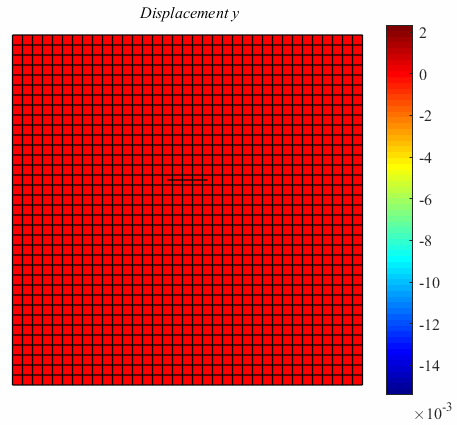Example 17: Crack propagation of rock under combined compression and shear loading